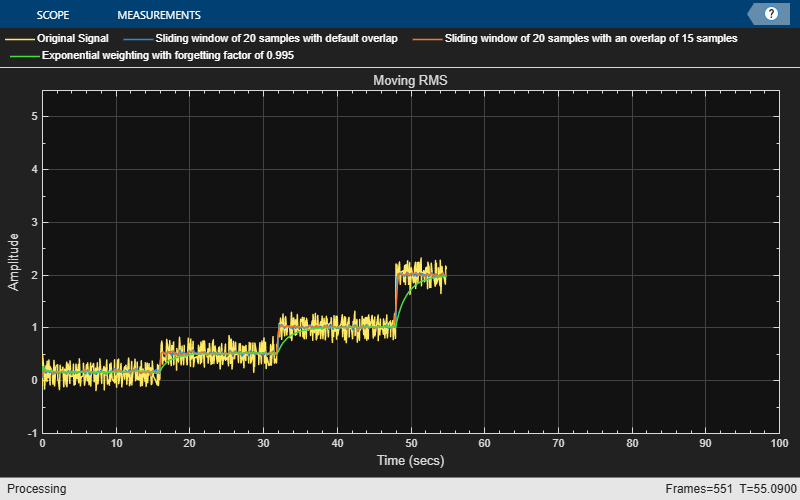# dsp.MovingRMS

Moving root mean square

## Description

The `dsp.MovingRMS` System object™ computes the moving root mean square (RMS) of the input signal along each channel, independently over time. The object uses either the sliding window method or the exponential weighting method to compute the moving RMS. In the sliding window method, a window of specified length is moved over the data, sample by sample, and the RMS is computed over the data in the window. In the exponential weighting method, the object squares the data samples, multiplies them with a set of weighting factors, and sums the weighed data. The object then computes the RMS by taking the square root of the sum. For more details on these methods, see Algorithms.

To compute the moving RMS of the input:

1. Create the `dsp.MovingRMS` object and set its properties.

2. Call the object with arguments, as if it were a function.

## Creation

### Syntax

``movRMS = dsp.MovingRMS``
``movRMS = dsp.MovingRMS(Len)``
``movRMS = dsp.MovingRMS(Name,Value)``

### Description

````movRMS = dsp.MovingRMS` returns a moving RMS object, `movRMS`, using the default properties.```
````movRMS = dsp.MovingRMS(Len)` sets the `WindowLength` property to `Len`.```

example

````movRMS = dsp.MovingRMS(Name,Value)` specifies additional properties using `Name,Value` pairs. Unspecified properties have default values.Example: ```movRMS = dsp.MovingRMS('Method','Exponential weighting','ForgettingFactor',0.9);``````

## Properties

expand all

Unless otherwise indicated, properties are nontunable, which means you cannot change their values after calling the object. Objects lock when you call them, and the `release` function unlocks them.

If a property is tunable, you can change its value at any time.

Moving RMS method, specified as `'Sliding window'` or `'Exponential weighting'`.

• `'Sliding window'` — A window of length specified by `SpecifyWindowLength` is moved over the input data along each channel. For every sample the window moves by, the object computes the RMS over the data in the window.

• `'Exponential weighting'` — The object multiplies the squares of the samples with a set of weighting factors. The magnitude of the weighting factors decreases exponentially as the age of the data increases, never reaching zero. To compute the RMS, the algorithm sums the weighted data, and takes a square root of the sum.

For more details on these methods, see Algorithms.

Flag to specify a window length, specified as a scalar Boolean.

• `true` — The length of the sliding window is equal to the value you specify in the `WindowLength` property.

• `false` — The length of the sliding window is infinite. In this mode, the RMS is computed using the current sample and all past samples.

#### Dependencies

This property applies when you set `Method` to ```'Sliding window'```.

Length of the sliding window in samples, specified as a positive scalar integer.

#### Dependencies

This property applies when you set `Method` to `'Sliding window'` and `SpecifyWindowLength` to `true`.

Data Types: `single` | `double` | `int8` | `int16` | `int32` | `int64` | `uint8` | `uint16` | `uint32` | `uint64`

Exponential weighting factor, specified as a positive real scalar in the range (0,1].

A forgetting factor of 0.9 gives more weight to the older data than does a forgetting factor of 0.1. A forgetting factor of 1.0 indicates infinite memory. All the past samples are given an equal weight.

Since this property is tunable, you can change its value even when the object is locked.

Tunable: Yes

#### Dependencies

This property applies when you set `Method` to `'Exponential weighting'`.

Data Types: `single` | `double`

## Usage

### Syntax

``y = movRMS(x)``

### Description

example

````y = movRMS(x)` computes the moving RMS of the input signal, `x`, using either the sliding window method or exponential weighting method.```

### Input Arguments

expand all

Data input, specified as a vector or a matrix. If `x` is a matrix, each column is treated as an independent channel. The moving RMS is computed along each channel. The object also accepts variable-size inputs. Once the object is locked, you can change the size of each input channel, but you cannot change the number of channels.

Data Types: `single` | `double`
Complex Number Support: Yes

### Output Arguments

expand all

Moving RMS of the input signal, returned as a vector or a matrix.

Data Types: `single` | `double`
Complex Number Support: Yes

## Object Functions

To use an object function, specify the System object as the first input argument. For example, to release system resources of a System object named `obj`, use this syntax:

`release(obj)`

expand all

 `step` Run System object algorithm `release` Release resources and allow changes to System object property values and input characteristics `reset` Reset internal states of System object

## Examples

collapse all

Compute the moving RMS of a noisy square wave signal with varying amplitude using the `dsp.MovingRMS` object.

Initialization

Set up `movrmsWin` and `movrmsExp` objects. `movrmsWin` uses the sliding window method with a window length of 20. `movrmsExp` uses the exponential weighting method with a forgetting factor of 0.995. Create a time scope for viewing the output.

```FrameLength = 10; Fs = 100; movrmsWin = dsp.MovingRMS(20); movrmsExp = dsp.MovingRMS('Method','Exponential weighting',... 'ForgettingFactor',0.995); scope = timescope('SampleRate',Fs,... 'TimeSpanOverrunAction','Scroll',... 'TimeSpanSource','Property',... 'TimeSpan',100,... 'ShowGrid',true,... 'YLimits',[-1.0 5.5]); title = 'Sliding Window RMS (blue) and Exponentially Weighted RMS (red)'; scope.Title = title; ```

Compute the RMS

Generate a noisy square wave signal. Vary the amplitude of the square wave after a given number of frames. Apply the sliding window method and the exponential weighting method on this signal. View the output on the time scope.

```count = 1; Vect = [1/8 1/2 1 2 3 4]; for index = 1:length(Vect) V = Vect(index); for i = 1:160 x = V + 0.1 * randn(FrameLength,1); y1 = movrmsWin(x); y2 = movrmsExp(x); scope([x,y1,y2]); end end ```## Algorithms

expand all

 Bodenham, Dean. “Adaptive Filtering and Change Detection for Streaming Data.” PH.D. Thesis. Imperial College, London, 2012.

Watch now